International
Tables for
Crystallography
Volume F
Crystallography of biological macromolecules
Edited by M. G. Rossmann and E. Arnold

International Tables for Crystallography (2006). Vol. F, ch. 25.2, pp. 697-698

## Section 25.2.1.5. Structure-factor and phase calculations

W. Fureya*

#### 25.2.1.5. Structure-factor and phase calculations

| top | pdf |

Several methods are used for structure-factor and phasing calculations depending on the nature of the model and how the results will be used. The methods available in the package are described below.

#### 25.2.1.5.1. By heavy-atom or anomalous-scattering methods

| top | pdf |

Phasing by heavy-atom-based methods (isomorphous replacement and/or anomalous scattering) begins when one or more scaled' data sets are input to the program PHASIT (batch). User-specified rejection criteria are first applied to each data set, and structure factors corresponding to the heavy-atom or anomalous-scatterer substructure are computed from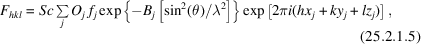where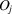is the occupancy,is the (possibly complex) scattering factor,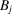is the isotropic temperature parameter and,,are the fractional coordinates of the jth atom. Sc is a scale factor relating the calculated structure factor (absolute scale) to the scale of the observed data. The summation is taken over all heavy atoms or anomalous scatterers in the unit cell. Alternatively, anisotropic temperature parameters can be used for each atom if desired. A subset of reflections comprising all centric data (plus the largest 25% of the isomorphous or anomalous differences if there are insufficient centric data) is selected and used to estimate Sc by a least-squares fit to the observed differences. Initial estimates of the standard error' E (expected lack of closure) are determined from this subset as a function of F magnitude, treating centric and acentric data separately. SIR (single isomorphous replacement) or SAS (single-wavelength anomalous scattering) phase probability distributions are given by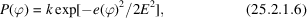where the lack of closure is defined by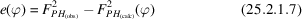for isomorphous-replacement data and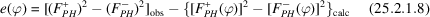for anomalous-scattering data, with theand − superscripts denoting members of a Bijvoet pair, and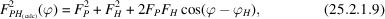with ϕ denoting the protein phase, andand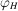denoting the heavy-atom structure-factor amplitude and phase, respectively. The distributions, however, are cast in the A, B, C, D form (Hendrickson & Lattman, 1970). After all input data sets are processed in this manner, the individual phase probability distributions for common reflections are combined via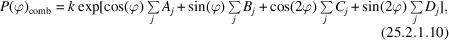with k as a normalization constant and the sums taken over all contributing data sets. The resulting combined distributions are then integrated to yield a centroid phase and figure of merit for each reflection. The standard error estimates, E, as a function of structure-factor magnitude are then updated for each data set, this time using all reflections and a probability-weighted average over all possible phase values for the contribution from each reflection (Terwilliger & Eisenberg, 1987a,b). With these updated standard error estimates, the individual SIR and/or SAS phase probability distributions are recomputed for all reflections and combined again to yield an improved centroid phase and figure of merit for each reflection. The resulting phases, figures of merit and probability distribution information are then available for use in map calculations or for further parameter or phase refinement. This method is used to produce MIR (multiple isomorphous replacement), SIRAS (single isomorphous replacement with anomalous scattering) MIRAS (multiple isomorphous replacement with anomalous scattering) and MAD phases as well as other possible phase combinations.

#### 25.2.1.5.2. Directly from atomic coordinates

| top | pdf |

Structure-factor amplitudes and phases for a macromolecular structure can be computed directly from atomic coordinates corresponding to a tentative model with the programs PHASIT and GREF (both run as batch processes). This allows one to obtain structure-factor information from an input model typically derived from a partial chain trace or from a molecular-replacement solution. Equation (25.2.1.5)is used, but this time the sum is taken over all known atoms in the cell, and the scale factor is refined by least squares against the native amplitudes rather than against the magnitudes of isomorphous or anomalous differences. The computed structure factors may be used directly for map calculations, including `omit' maps, or for combination with other sources of phase information. One can output probability distribution information for the calculated phases, if desired, as well as coefficients for various Fourier syntheses, including those using sigma_A weighting (Read, 1986) for the generation of reduced-bias native or difference maps.

#### 25.2.1.5.3. By map inversion

| top | pdf |

For the purpose of improving phases by density-modification methods, such as solvent flattening, negative-density truncation and/or NC symmetry averaging, one must compute structure factors by Fourier inversion of an electron-density map rather than from atomic coordinates. The program MAPINV (batch) is a companion program to FSFOUR and carries out this inverse Fourier transform. It accepts a full-cell map in FSFOUR format and inverts it to produce amplitudes and phases for a selected set of reflections when given the target range of Miller indices. A variable-radix 3D fast Fourier transform algorithm is used. Optionally, the program can modify the density prior to inversion by truncation below a cutoff and/or by squaring the density values. Other types of density modification are handled by different programs in the package and are carried out prior to running MAPINV. The indices, calculated amplitude and phase are written to a file for each target reflection.

### References

Hendrickson, W. A. & Lattman, E. E. (1970). Representation of phase probability distributions for simplified combination of independent phase information. Acta Cryst. B26, 136–143.Google Scholar
Read, R. J. (1986). Improved Fourier coefficients for maps using phases from partial structures with errors. Acta Cryst. A42, 140–149.Google Scholar
Terwilliger, T. C. & Eisenberg, D. (1987a). Isomorphous replacement: effects of errors on the phase probability distribution. Acta Cryst. A43, 6–13.Google Scholar
Terwilliger, T. C. & Eisenberg, D. (1987b). Isomorphous replacement: effects of errors on the phase probability distribution. Erratum. Acta Cryst. A43, 286.Google Scholar